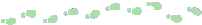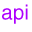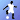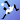## Composite Names

Recall that a composite name is a name that spans multiple naming systems. Here's an example of a composite name:
```cn=homedir,cn=Jon Ruiz,ou=People/tutorial/report.txt
```
This is the string representation of a composite name that contains two parts: an LDAP name, "cn=homedir,cn=Jon Ruiz,ou=People", and a filename, "tutorial/report.txt". When you pass this string to a Contextmethod, such as lookup(), in the namespace set up as recommended by this tutorial (see the Preparationslesson), the method will resolve through the LDAP directory to the file system and return the target object (a file). The mechanics of how this is accomplished by the underlying service provider implementations are described in the Federationlesson.

Here's an example.

```File f = (File)ctx.lookup(
"cn=homedir,cn=Jon Ruiz,ou=People/tutorial/report.txt");
```

#### String Representation

A composite name is made up of components. You can think of a component as a segment of the composite name that belongs to a single naming system. Each component is separated by a forward slash character ("/").

For example, the name

```cn=homedir,cn=Jon Ruiz,ou=People/tutorial/report.txt
```
has three components:
```cn=homedir,cn=Jon Ruiz,ou=People
tutorial
report.txt
```
The first component belongs to the LDAP namespace, and the second two belong to the file system namespace. As you can see from this example, multiple components from the same namespace are allowed ("tutorial" and "report.txt" are both from the file system namespace), but one component cannot span more than one namespace. (See the discussion on this in the Federationlesson.)

In addition to the forward slash character, the composite name syntax allows three other special characters: the backslash character ("\"), the single quotation character ("'"), and the double quotation character ("""). The slash, backslash, and quotation characters are called meta characters, which means they have special meanings when they occur in a composite name. The backslash character is the escape character. When the escape character precedes a meta character, the meta character is treated literally and not interpreted according to its special meaning. For example, in the string a\/b, the forward slash character is escaped by the backslash character and therefore is not treated as a composite name component separator. See the Handling Special Characters section of this lesson for a more detailed discussion of meta characters.

The quotation characters are provided so that you can use the meta characters within a composite name component without having to escape them. When a component is quoted, its first and last characters must be (the same) quotation characters. A single quotation character must be matched by a single quotation character, and a double quotation character must be matched by a double quotation character. Here are three examples of the same component written by using escapes and quotation characters.

```a\/b\/c\/d
"a/b/c/d"
'a/b/b/d'
```
Two different quotation characters are permitted so as to allow quoting when a quotation character already exists in the component. For example, a component containing a double quotation character can be represented as either \"ab or '"ab'.

A composite name can be empty; that is, it contains zero components. An empty composite name is represented by the empty string.

A composite name component can be empty, that is, it contains an empty string. A leading component separator (the composite name string begins with a separator) denotes a leading empty component. A trailing component separator (the composite name string ends with a separator) denotes a trailing empty component. Adjacent component separators denote an empty component. Here are examples of each.

```/abc
abc/
abc//xyz
```

See the discussion in the Handling Special Characters section of this lesson about how best to accommodate special characters in a name that conflicts with the JNDI composite name syntax.

#### The CompositeName Class

The CompositeNameclass is the structural form of a composite name. The constructoraccept a string representation of a composite name and parses it into components according to the composite name syntax.

Here's an example that uses the constructor to parse a composite name and then prints its components.

```String name = // Composite name to parse
try {
CompositeName cn = new CompositeName(name);
System.out.println(cn + " has " + cn.size() + " components: ");
for (int i = 0; i < cn.size(); i++) {
System.out.println(cn.get(i));
}
} catch (InvalidNameException e) {
System.out.println("Cannot parse name: " + name);
}
```
Running this example with an input of a/b/c produces the following results.
```a/b/c has 3 components:
a
b
c
```

The CompositeName class contains methods to access components, to modify a CompositeName, to compare two CompositeNames for equality, and to get the string representation of a CompositeName.

#### Accessing Components of a Composite Name

Here are the methods that you can use to access components of a composite name:
get(int posn)getAll()getPrefix(int posn)getSuffix(int posn)clone()To retrieve the component at a particular position within a CompositeName, you use get(). The previous constructor example shows an example of its use.

getAll() returns all of the components of a CompositeName as an enumeration. You iterate through the enumeration to get each component. The constructor example can be rewritten using an enumeration, as shown next.

```try {
CompositeName cn = new CompositeName(name);
System.out.println(cn + " has " + cn.size() + " components: ");
for (Enumeration all = cn.getAll(); all.hasMoreElements();) {
System.out.println(all.nextElement());
}
} catch (InvalidNameException e) {
System.out.println("Cannot parse name: " + name);
}
```

You can also get a CompositeName's suffix or prefix as a CompositeName instance. Here's an example that gets the suffix and prefix of a composite name.

```CompositeName cn = new CompositeName("one/two/three");
Name suffix = cn.getSuffix(1);  // 1 <= index < cn.size()
Name prefix = cn.getPrefix(1);  // 0 <= index < 1
```
When you run this program, it generates the following output.
```two/three
one
```
To make a copy of a CompositeName, you use clone().

#### Modifying a Composite Name

Following are the methods that you can use to modify a composite name:
add(String comp)add(int posn, String comp)addAll(Name comps)addAll(Name suffix)addAll(int posn, Name suffix)remove(int posn)After creating a CompositeName instance, you can add and remove components from it. Here's an example that appends a CompositeName to an existing CompositeName, adds components to the front and the end, and removes the second component.

```CompositeName cn = new CompositeName("1/2/3");
CompositeName cn2 = new CompositeName("4/5/6");
System.out.println(cn.remove(1));             // 1
System.out.println(cn);			      // abc/2/3/4/5/6/xyz
```

#### Comparing a Composite Name

Following are the methods that you can use to compare two composite names:
compareTo(Object name)equals(Object name)endsWith(Name name)startsWith(Name name)isEmpty()You can use compareTo() to sort a list of CompositeName instances. Here's an example that uses compareTo() to implement the Bubble Sort algorithm.
```private static void sort(CompositeName[] names) {
int bound = names.length-1;
CompositeName tmp;

while (true) {
int t = -1;
for (int j=0; j < bound; j++) {
int c = names[j].compareTo(names[j+1]);
if (c > 0) {
tmp = names[j];
names[j] = names[j+1];
names[j+1] = tmp;
t = j;
}
}
if (t == -1) break;
bound = t;
}
}
```
equals() lets you determine whether two CompositeNames are syntactically equal. Two CompositeNames are equal if they both have the same (case-exact matched) components in the same order.

With startsWith() and endsWith(), you can learn whether a CompositeName starts or ends with another CompositeName; that is, whether a CompositeName is a suffix or prefix of another CompositeName.

The convenience method isEmpty() enables you to determine whether a CompositeName has zero components. You can also use the expression size() == 0 to perform the same check.

Here are examples of using some of these comparison methods.

```CompositeName one = new CompositeName("cn=fs/o=JNDITutorial/tmp/a/b/c");
CompositeName two = new CompositeName("tmp/a/b/c");
CompositeName three = new CompositeName("cn=fs/o=JNDITutorial");
CompositeName four = new CompositeName();

System.out.println(one.equals(two)); 	    // false
System.out.println(one.startsWith(three));  // true
System.out.println(one.endsWith(two));      // true
System.out.println(one.startsWith(four));   // true
System.out.println(one.endsWith(four));     // true
System.out.println(one.endsWith(three));    // false
System.out.println(one.isEmpty());	    // false
System.out.println(four.isEmpty());	    // true
System.out.println(four.size() == 0);	    // true
```

#### Getting the String Representation

Following is the method that you can use to get the string representation of a composite name:
`toString()`
When you use the CompositeName constructor, you supply the string representation of a composite name and get back a CompositeName instance. To do the reverse, that is, to get the string representation of a CompositeName instance, you use toString(). The result of toString() can be fed back to the constructor to produce a CompositeName instance that is equal to the original CompositeName instance. Here's an example.
```CompositeName cn = new CompositeName(name);
String str = cn.toString();
System.out.println(str);
CompositeName cn2 = new CompositeName(str);
System.out.println(cn.equals(cn2));		// true
```

#### CompositeName as an Argument to Context Methods

A CompositeName instance passed to methods in the Contextand DirContextinterfaces is treated as a composite name. Here is an example that looks up an object by first creating a CompositeName instance that represents its name.
```// Create the initial context
Context ctx = new InitialContext(env);

// Parse the string name into a CompositeName
Name cname = new CompositeName(
"cn=homedir,cn=Jon Ruiz,ou=people/tutorial/report.txt");

// Perform the lookup using the CompositeName
File f = (File) ctx.lookup(cname);
```